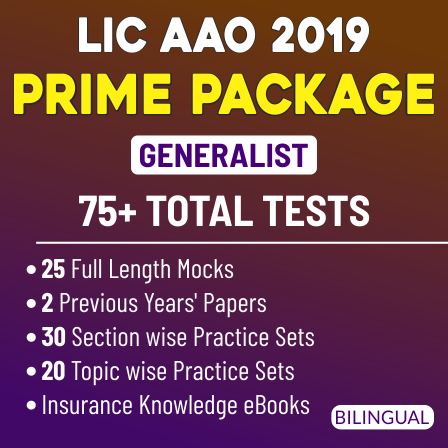# Study Notes & Short Tricks: Inequalities part-1

Dear Students,

As per today’s scenario in the Banking exam even in other exams like SSC, it has become important that to solve a particular problem quickly you have to know the concept behind the question and also the way how to solve that problem quickly. Keeping in mind that thing we are providing here some important study notes and short tricks of various topics of Reasoning Ability so that a student can attempt more questions in less time (especially in Banking and SSC exams) and can make a higher probability of being selected. Best of hard work to all students. Keep using Bankersadda.

Inequalities is one of the topic where you can get full marks very easily. It is a common topic for all competitive exams. We can expect 3 to 5 questions from Inequality.
Before discussing details for solving Inequalities questions, lets check the meaning of certain symbols in below table:–
To check the different relationship, we are presenting some different statement and conclusions in below table. From given below table you will get clear concept of relationship between two letters.

Priority of Symbols :–
1) >  ≥ =
For ex- If    T>P≥Q=R
Then, T> Q and T>R

2) <  ≤ =
For ex- If    W<X≤V=Y
Then, W<Y and W<V

3) >  < (No relation)
For ex- If Q>K<L
Then there will be no relation between Q and L.

4) >  ≤ (No relation)
For ex- If O>J≤H
Then there will be no relation between O and H.

5) <  > (No relation)
For ex- If F<E>Q
Then there will be no relation between F and Q.

6) <  ≥ (No relation)
For ex- If D<S≥Z
Then there will be no relation between D and Z.

Either- or case :¬– In equality it is very important condition. Mostly students make mistakes in this condition. For clear concept we are giving example of “either-or”
1st condition for “either-or” is both conclusions should be wrong.
2nd condition is that variables of both conclusions should be same.
Eg. :–
Statement:  A≥B=C
Conclusion: (a) A > C (b) A = C
(û            (û)
In above example, relation between A and C is A≥C. But both the conclusions are wrong and both have same variables. And by combining both conclusions you will get the actual relation between A and C which comes from statement.

2. Statement: P=Q≥R≥S=T
Conclusion I:  (a)P>T                      (b)P=T
From the above statement it is clear that P is either  equals to T or P is greater than T ,So individually both the conclusions are wrong but by combining them we will get that P is either greater than or equals to T (P≥T).

Conclusion II: (a) Q>S                           (b) Q=S
Similarly from the above statement for conclusion II we can see that there is an either / or case between Q and S, So Q either will be greater than or equals to S.

Complicated case of “Either-or” :
Statement:  H≥M≤V=K
Conclusions: (1) H<K (2) H≥K
(û)                 (û

In the above statement we cannot find the relation between H and K. There may be three possibilities of relation between H and K.
i.e. (a) H>K (b) H<K (c) H=K
And we are getting all possibilities by combining both conclusions. So, this is also one case of “Either-or”.

Statement: F<T≤N,F>S,M≤T<G
Conclusions: I.M≥S        II. S>M

In the above question by combining the statements together we get   S<F<T≥M. So we cannot find the relation between M and S. As there can be three possible cases: M is either greater, lesser or equals to S. In conclusions I and II we can find all the three possible cases so the answer will be either conclusion I or II follow.

Statement: L≥K<E≥A>F≥B
Conclusions: I.L<B             II.B≤L

This is another example showing that no direct relation is found between B and L and all the three possible conditions as L>B, L<B or L=B can be there. So the answer will be either conclusion I or II follow.
Questions based on inequality are of two types:–
(1) Direct Inequality
(2) Indirect Inequality
This is Part I of handy notes……..next part will come in next month edition…….So stay connected with Competition Power…….